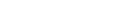#### Latest News

#### Society of Dairy Technology (SDT) webinar on Thursday the 17th of November.

In Northern Europe and North America there is relatively little use made of whole milk powder (WMP) in commercial  ice cream manufacture. However small scale ice cream or gelato manufacturers sometimes use these ingredients.  In Vietnam and other Asian countries ice cream is frequently made from whole milk powder and cream.

To the best of my knowledge there has been no information published on how to calculate the weights of cream and whole milk powder required to make ice cream mixes to a product specification. However the basic principles of mix calculation have been well described.

The aim of this tutorial is to explain the calculations required to determine the weight of  cream and WMP in ice cream mixes. The significance of being able to undertake these calculations and an explanation of the concept of a balanced ice cream mix has been explained previously. We will start by first defining the mix specification that is required.

Target mix composition:

8% fat, 11.2% milk-solids-not-fat (MSNF), 13% sugar, 0.3% emulsifier, 0.3% stabiliser.

Next we will define the ingredients that are to be used.

Ingredients:Cream (40% fat), WMP (26% fat), sugar, emulsifier and stabiliser. Note that the MSNF concentration of the cream and the WMP have not been given and will have to be calculated.

I will now explain how the mix ingredients can be calculated assuming a 100 kg mix.

Solution:

The sugar, emulsifier and stabiliser ingredient weights are calculated as follows:

1.            Sugar:                   13/100 x 100 = 13 kg

2.            Emulsifier:            0.3/100 x 100= 0.3 kg

3.            Stabiliser:            0.3/100 x 100= 0.3 kg

The weights of cream and WMP required can be obtained using several methods, one of the easier understood methods is to express the MSNF and fat present in the mix, and in the contributing components, using a  2-variable simultaneous equation system.

Before we write the equations we first need to calculate the MSNF in the cream and the WMP.

The MSNF in 100 kg of cream of is obtained by subtracting the weight of fat present from 100 and multiplying by 0.09 to obtain the MSNF.

= 100-40*0.09

=5.4 kg MSNF

=5.4% MSNF

The MSNF in 100 kg of WMP is obtained by subtracting the fat and moisture values from 100.

= 100- (26 + 3)

=71 kg MSNF

= 71% MSNF

We will now write the two variable equations.

Let x= cream required (kg)

Let y= WMP required (kg)

Firstly we will undertake a MSNF balance.

5.4 x   +   71 y = 11.2
100          100

Next we will undertake a fat balance.

40 x   +   26 y = 8
100          100

Students should solve x and y in the normal way showing all the calculation steps to obtain  full marks in assignments.

However, in product development situations where lots of calculations are required it advantageous to use a spreadsheet programme  to solve the equations.  Cramer’s rule can easily be adapted for use with spreadsheets.

Cramer’s rule can be illustrated using the two equations below that we want to solve for x and y.

Equation 1:  ax+by=e

Equation 2:  cx+dy=f

It can be shown that

A screen shot of an Excel spreadsheet using Cramer’s rule to solve x and y is shown below. It is apparent that 10.25 (x)  kg of WMP and 14.99 (y) kg of cream are required.The final ingredient, water is calculated by subtracting all the other ingredients from 100.

Water= 100- (cream +WMP +sugar+ emulsifier + stabiliser)

= 100- (10.25 +14.99  + 13 +0.3 +0.3)

= 61.15 kg

We now have all the weights of the ingredients but we need to check our calculations and make certain that the weights of cream and WMP contribute the correct weights of fat and MSNF.

This is done using a simple table as shown below.It is clear that the masses of all the ingredients sum to 100 and that the MSNF and fat components also balance.

Using the information above it is relatively easy to produce a spreadsheet to calculate a range of mixes using WMP and cream. An additional table can be added to enable costs to be calculated. The table for solving simultaneous equations can easily be modified to enable more complex mix calculations to be undertaken.

Cost can be reduced by producing ice cream using vegetable fat/cream blends. Spreadsheets can easily be adapted to enable cream / vegetable fat mixes to be configured. An example of an Excel spreadsheet configured to solve mix ingredient problems can be accessed here. This is for illustrative purposes only. Working spreadsheets are available and can be downloaded by making a small contribution to the site running costs. This tutorial along with the extensive range of worked examples on Professor Doug Goff's website should enable students and product development technologists to undertake virtually any type of ice cream mix calculation for "free".